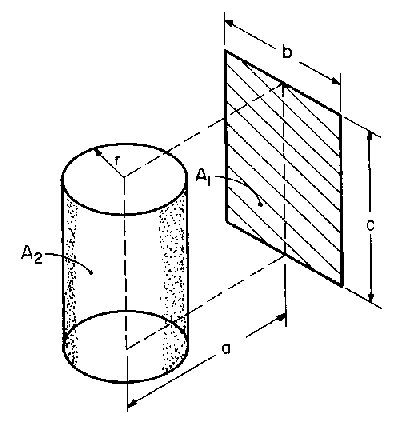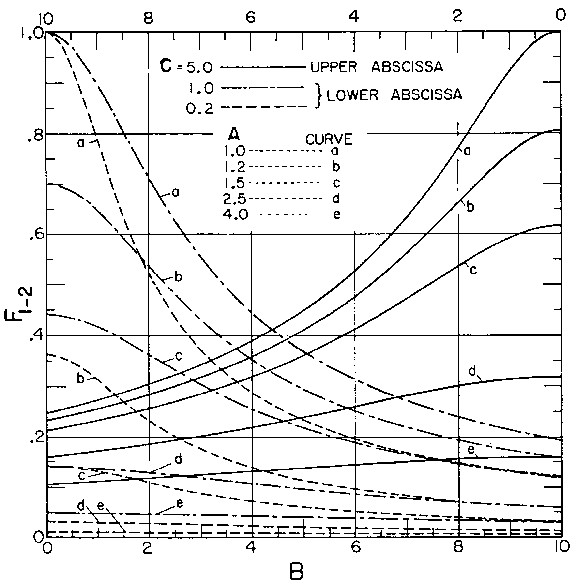# SECTION C Factors From Finite Areas to Finite Areas

## C-74: Finite-length cylinder to rectangle with two edges parallel to cylinder axis and of length equal to cylinder.Definitions:

 A = a/r; B = b/r;  C = c/r

Graphical Results: